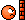# Unitary vector commuting with Hamiltonian and effect on system

happyparticle
Homework Statement:
U unitary operator that commute with H.
##| \psi_n \rangle## an eigenstate of H with eigenvalue ##E_n##
##| \phi_n \rangle = U | \psi_n \rangle##

Thus,
##| \phi_n \rangle = \sum_i \alpha_i |\psi_n^i \rangle##
Relevant Equations:
##| \phi_n \rangle = \sum_i \alpha_i |\psi_n^i \rangle##
Hi,

I'm not sure to understand what ##| \phi_n \rangle = \sum_i \alpha_i |\psi_n^i## means exactly or how we get it.
From the statement, I understand that ##[U,H] = 0## and ##H|\psi_n \rangle = E_n|\psi_n \rangle##

Also, a linear combination of all states is also an solution.

If U commutes with H then they have the same eigenstates (and same eigenvalues ?)
Thus, ##U|\psi_n \rangle = E_n | \psi_n \rangle##

I have hard time to put all those things together or seeing what that really means.

Thank you

Is that the entire homework question you were given? How does the question define the ##|\psi^i_n\rangle## states?

I'm not sure if this is relevant, but,... if ##H## is the usual Hamiltonian operator, it's presumably Hermitian. There's a theorem that the eigenstates of an Hermitian operator span the Hilbert space, hence any other state can be expressed as a linear combination of ##H##'s eigenstates ##|\psi_n\rangle##. But in this, ##U## plays no role. Again, what are the ##|\psi^i_n\rangle## states??

You also have $$H |\phi_n \rangle ~=~ HU |\psi_n \rangle ~=~ UH |\psi_n \rangle ~=~ U E_n |\psi_n\rangle ~=~ E_n |\phi_n \rangle ~,$$but I'm not sure how that helps here. Are the eigenstates of ##H## degenerate? I.e., more than 1 eigenstate with the same ##E_n## eigenvalue? Is that what the ##|\psi^i_n\rangle## denote?

•vanhees71
happyparticle
Is that the entire homework question you were given? How does the question define the ##|\psi^i_n\rangle## states?
Hi, yeah this is the entire homework question and the only thing we know about ##
|\psi^i_n\rangle
##
is that ##
| \phi_n \rangle = \sum_i \alpha_i |\psi_n^i \rangle
##

Where i is the degree of degeneracy

Hi, yeah this is the entire homework question and the only thing we know about ##
|\psi^i_n\rangle
##
is that ##
| \phi_n \rangle = \sum_i \alpha_i |\psi_n^i \rangle
##

Where i is the degree of degeneracy
You omitted that (crucial) last sentence from your original problem statement. You also didn't include what the question was actually asking.That's why the PF homework guidelines urge you to show the whole, unedited problem statement.

(I'm sure that being a "happy" particle is a pleasant state of mind, but maybe you should lay off the weed while attempting physics study?)

Anyway, now it's easy. Since ##|\phi_n\rangle## must be an eigenstate of ##H## with eigenvalue ##E_n##, it must be a linear combination of the ##|\psi_n^i \rangle##.

•vanhees71
happyparticle
You omitted that (crucial) last sentence from your original problem statement.
I thought it was implied, sorry. Not because I thought you should know, but I thought that the form ##
| \phi_n \rangle = \sum_i \alpha_i |\psi_n^i \rangle## implies that i is the degree of degeneracy.
You also didn't include what the question was actually asking.
I have to show exactly this##
| \phi_n \rangle = \sum_i \alpha_i |\psi_n^i
##
However, as I said I'm not sure how to do with what I know or what the equation above means exactly.

I'm not sure to understand why the i power ##
|\psi_n^i \rangle
##

I'm not sure to understand why the i power ##|\psi_n^i \rangle##
The "i" is not a power, just an upstairs index to distinguish the various degenerate eigenstates.

•vanhees71
Gold Member
2022 Award
You omitted that (crucial) last sentence from your original problem statement. You also didn't include what the question was actually asking.That's why the PF homework guidelines urge you to show the whole, unedited problem statement.
Also note that carefully reading of the problem text is the 0th step to the solution!

•strangerep
happyparticle
Anyway, now it's easy. Since |ϕn⟩ must be an eigenstate of H with eigenvalue En, it must be a linear combination of the |ψni⟩.
I spent a big chuck of the weekend to convince myself, but I'm not sure to understand.
##U|\psi_n \rangle = E_n|\psi_n\rangle = | \phi_n \rangle##
I think with that I can say that ##| \phi_n \rangle## is an eigenvector of H, but I would say with eigenvalue 1.

Furthermore, I don't see why we can say that ##| \phi_n \rangle## must be a linear combination of ##|\psi_n^i\rangle##

I spent a big chuck of the weekend to convince myself, but I'm not sure to understand.
##U|\psi_n \rangle = E_n|\psi_n\rangle = | \phi_n \rangle##
The middle bit looks wrong. You are given that ##H|\psi_n \rangle = E_n|\psi_n\rangle##, but this does not imply ##U|\psi_n \rangle = E_n|\psi_n\rangle##.

I think with that I can say that ##| \phi_n \rangle## is an eigenvector of H,
Not the way you tried to do it. I have already given you the correct method in my post #2. And its eigenvalue is ##E_n##, not 1. This was also shown in my post #2.

Furthermore, I don't see why we can say that ##| \phi_n \rangle## must be a linear combination of ##|\psi_n^i\rangle##
After you have proven that ##|\phi_n \rangle## is an eigenstate of ##H## with eigenvalue ##E_n##, then...

Suppose there was only 1 eigenstate of ##H## (i.e., suppose ##H## were not degenerate). Then you could invoke the theorem that the eigenstates of an Hermitian operator span the Hilbert space, and are mutually orthogonal;-- that would be enough to prove ##|\phi_n\rangle = |\psi_n\rangle##.

Even in the degenerate case it is still true that the eigenstates with different eigenvalues are orthogonal. Therefore, since any state can be expressed as a sum over all ##H##'s eigenstates, we can express ##|\phi_n\rangle## as such a sum. But ##|\phi_n\rangle## must be orthogonal to all ##H##'s eigenstates that don't have eigenvalue ##E_n##, hence those eigenstates cannot appear in the sum for ##|\phi_n\rangle##. That leaves only the ##|\psi_n^i\rangle## states in the sum.

•happyparticle, PeroK and vanhees71